### 起亚(进口) 嘉华(进口)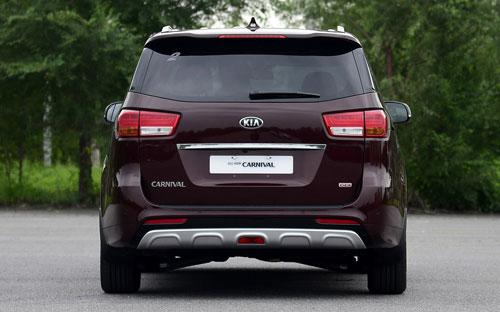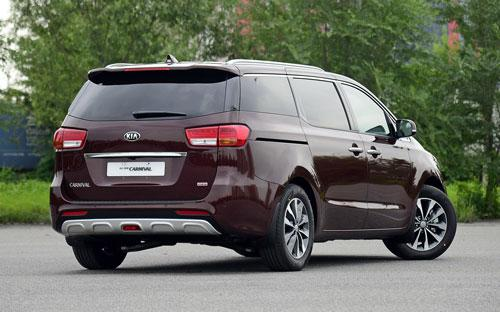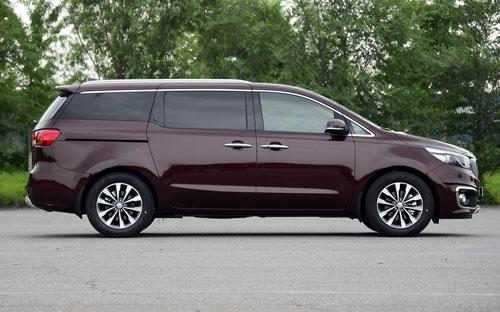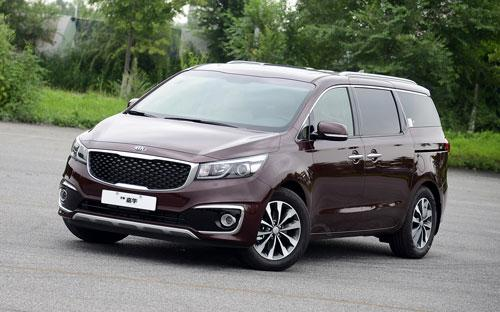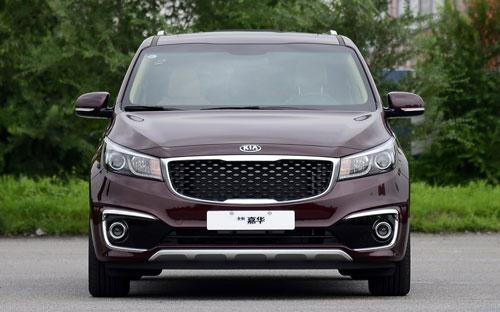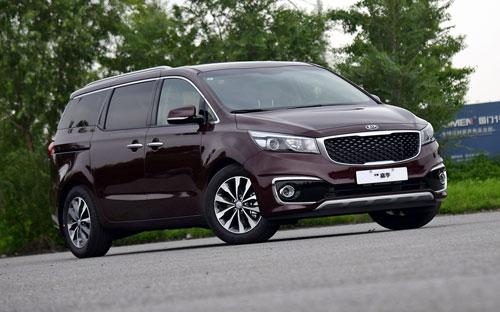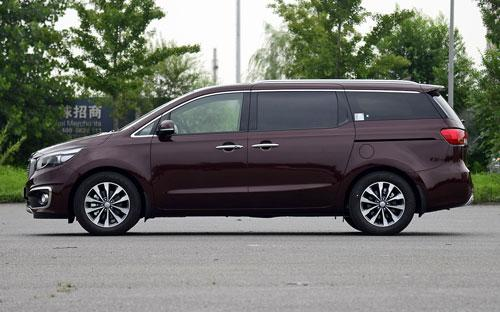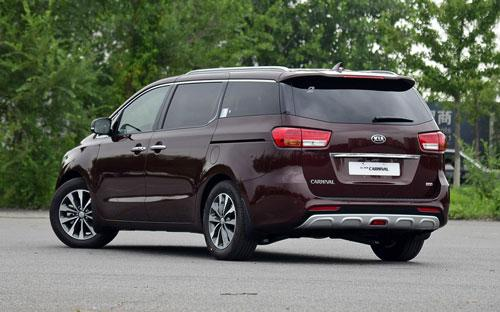9 种颜色可选2015款最低售价：26.98 万元起

5115(mm)1985(mm)1790(mm)##### 配置亮点：
• 胎压监测装置

• ISOFIX儿童座椅接口

• 车身稳定控制(ESC/ESP/DSC等)

• 电动天窗

• 定速巡航

• 后倒车雷达

• 真皮座椅

• GPS导航系统

• 氙气大灯

• 后视镜加热

• 提交
2015款 2.2T 旗舰版 (96张)
• 2015款 2.2T 旗舰版 (96张)
• 2015款 3.3GDI 豪华版 (287张)
• 起亚(进口) 嘉华(进口) 绕车实拍• 起亚(进口) 嘉华(进口) 在售车型

排量 车型 厂商指导价 本地最低报价 购车工具
3.3L
3.3GDI 舒适版 6挡手自一体
30.98万
30.98万

3.3GDI 豪华版 6挡手自一体
33.98万
3.3GDI 旗舰版 6挡手自一体
38.98万
38.98万

2.2T
2.2T 舒适版 柴油 6挡手自一体
26.98万
26.98万

2.2T 豪华版 柴油 6挡手自一体
29.68万
2.2T 旗舰版 柴油 6挡手自一体
33.98万

起亚(进口) 嘉华(进口) 经销商

查看更多 >>

### 起亚(进口) 嘉华(进口) 动力加速

嘉华(进口) 0-100公里加速时间分布在 0.0-秒 属于 超跑级

动力级别 加速时间 车型

起亚(进口) 嘉华(进口) 视频

起亚(进口) 嘉华(进口) 新闻资讯

# 动力感受轻盈 试驾起亚全新嘉华

评测 超过7355次关注

全新嘉华在动力方面有3.3L自然吸气发动机和2.2T柴油发动机，与两款不同发动机搭配的都是6AT变速箱。我今天试驾的就是搭载3.3L自然吸气发动机的车型，这台3.3L发动机...

# 起亚新嘉华正式上市 售26.98-38.98万元

新闻 超过7787次关注

起亚官方宣布，全新嘉华正式上市，此次上市的新嘉华共有2个排量6款车型，售价区间为26.98-38.98万元。

# 同级优势明显 起亚全新嘉华到店体验

导购 超过11738次关注

把全新嘉华放到同级别市场中去看，它的优势是相当明显的，身材尺寸跟“霸主”GL8不相上下，如果你对乘坐空间和后备厢空间有硬性要求，那么除了别克GL8以及相对昂贵...

# 7月30日上市 起亚全新嘉华详细配置曝光

新闻 超过7313次关注

起亚全新嘉华车搭载2.2T柴油发动机和3.3L汽油发动机发动机，提供舒适版、豪华版和旗舰版三种不同的配置搭配。

# 内饰豪华 起亚全新嘉华将于7月30日上市

新闻 超过7299次关注

起亚全新嘉华将会于7月30日上市，新车将搭载3.3L汽油发动机和2.2L涡轮增压柴油发动机。

猜你喜欢

﻿
• 快速找车
• 选择品牌
• 选择品牌
• A  奥迪
• A  阿斯顿·马丁
• A  阿尔法·罗密欧
• B  宝沃
• B  布加迪
• B  巴博斯
• B  保时捷
• B  宾利
• B  奔驰
• B  宝马
• B  本田
• B  别克
• B  标致
• B  比亚迪
• B  宝骏
• B  北汽制造
• B  北汽新能源
• B  北汽幻速
• B  北汽威旺
• B  北京汽车
• B  奔腾
• B  北汽绅宝
• C  长安
• C  长安商用
• C  长城
• C  昌河
• D  大众
• D  道奇
• D  DS
• D  东南
• D  东风风神
• D  东风风行
• D  东风小康
• D  东风风度
• D  东风
• F  福特
• F  丰田
• F  菲亚特
• F  法拉利
• F  福田
• F  福迪
• F  福汽启腾
• G  观致
• G  广汽传祺
• G  广汽吉奥
• G  GMC
• H  红旗
• H  汉腾汽车
• H  哈弗
• H  哈飞
• H  海格
• H  海马
• H  华颂
• H  黄海
• H  华泰
• H  恒天
• J  吉利汽车
• J  捷豹
• J  Jeep
• J  江淮
• J  江铃
• J  金杯
• J  九龙
• J  金旅
• K  凯翼
• K  凯迪拉克
• K  克莱斯勒
• K  科尼塞克
• K  卡威
• K  开瑞
• L  路虎
• L  林肯
• L  劳斯莱斯
• L  兰博基尼
• L  雷克萨斯
• L  铃木
• L  雷诺
• L  理念
• L  力帆
• L  莲花汽车
• L  猎豹
• L  路特斯
• L  陆风
• M  马自达
• M  MG
• M  MINI
• M  玛莎拉蒂
• M  摩根
• M  迈凯轮
• N  纳智捷
• O  欧宝
• O  讴歌
• O  欧朗
• Q  奇瑞
• Q  起亚
• Q  启辰
• R  日产
• R  荣威
• R  瑞麒
• S  三菱
• S  斯威汽车
• S  萨博
• S  smart
• S  斯柯达
• S  斯巴鲁
• S  思铭
• S  双龙
• S  上汽大通
• S  双环
• T  特斯拉
• T  腾势
• W  沃尔沃
• W  五菱汽车
• W  五十铃
• W  威兹曼
• W  威麟
• X  现代
• X  雪佛兰
• X  雪铁龙
• X  西雅特
• Y  一汽
• Y  英菲尼迪
• Y  英致
• Y  依维柯
• Y  野马汽车
• Y  永源
• Z  众泰
• Z  中华
• Z  中兴
• Z  知豆
• 选择车系
• 选择车系
• 车型对比
• 选择品牌
• 选择品牌
• A  奥迪
• A  阿斯顿·马丁
• A  阿尔法·罗密欧
• B  宝沃
• B  布加迪
• B  巴博斯
• B  保时捷
• B  宾利
• B  奔驰
• B  宝马
• B  本田
• B  别克
• B  标致
• B  比亚迪
• B  宝骏
• B  北汽制造
• B  北汽新能源
• B  北汽幻速
• B  北汽威旺
• B  北京汽车
• B  奔腾
• B  北汽绅宝
• C  长安
• C  长安商用
• C  长城
• C  昌河
• D  大众
• D  道奇
• D  DS
• D  东南
• D  东风风神
• D  东风风行
• D  东风小康
• D  东风风度
• D  东风
• F  福特
• F  丰田
• F  菲亚特
• F  法拉利
• F  福田
• F  福迪
• F  福汽启腾
• G  观致
• G  广汽传祺
• G  广汽吉奥
• G  GMC
• H  红旗
• H  汉腾汽车
• H  哈弗
• H  哈飞
• H  海格
• H  海马
• H  华颂
• H  黄海
• H  华泰
• H  恒天
• J  吉利汽车
• J  捷豹
• J  Jeep
• J  江淮
• J  江铃
• J  金杯
• J  九龙
• J  金旅
• K  凯翼
• K  凯迪拉克
• K  克莱斯勒
• K  科尼塞克
• K  卡威
• K  开瑞
• L  路虎
• L  林肯
• L  劳斯莱斯
• L  兰博基尼
• L  雷克萨斯
• L  铃木
• L  雷诺
• L  理念
• L  力帆
• L  莲花汽车
• L  猎豹
• L  路特斯
• L  陆风
• M  马自达
• M  MG
• M  MINI
• M  玛莎拉蒂
• M  摩根
• M  迈凯轮
• N  纳智捷
• O  欧宝
• O  讴歌
• O  欧朗
• Q  奇瑞
• Q  起亚
• Q  启辰
• R  日产
• R  荣威
• R  瑞麒
• S  三菱
• S  斯威汽车
• S  萨博
• S  smart
• S  斯柯达
• S  斯巴鲁
• S  思铭
• S  双龙
• S  上汽大通
• S  双环
• T  特斯拉
• T  腾势
• W  沃尔沃
• W  五菱汽车
• W  五十铃
• W  威兹曼
• W  威麟
• X  现代
• X  雪佛兰
• X  雪铁龙
• X  西雅特
• Y  一汽
• Y  英菲尼迪
• Y  英致
• Y  依维柯
• Y  野马汽车
• Y  永源
• Z  众泰
• Z  中华
• Z  中兴
• Z  知豆
• 选择车系
• 选择车系
• 选择车型
• 选择车型
• 意见反馈